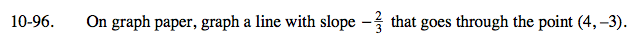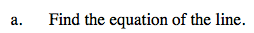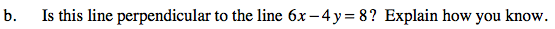### Home > AC > Chapter 10 > Lesson 10.2.5 > Problem10-96

10-96.Substitute in the known values for slope x, and y to find the y-intercept of this line.

Now solve this equation for b.The slope of a line (a) perpendicular to another line (b) is the opposite reciprocal of line (b)'s slope.
Solve this equation for y to find the slope and compare it to the slope of the line in part (a).